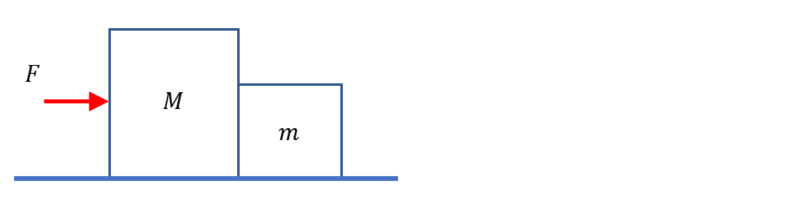Problem: Two boxes, one of mass M and one of mass m, lie next to each other. If a force F is applied on box M, as indicated in the figure, draw a free body diagram for each box, with the appropriate value for each force given in terms of known vairables (M, m, F, and g). Assume there is no friction along the surface.

FREE Expert Solution
80% (166 ratings)
Problem Details

Two boxes, one of mass M and one of mass m, lie next to each other. If a force F is applied on box M, as indicated in the figure, draw a free body diagram for each box, with the appropriate value for each force given in terms of known vairables (M, m, F, and g). Assume there is no friction along the surface.